# Online Gaussian Mixture Model Estimation¶

Online estimation of finite mixture models are implemented with Stochastic Expectation-Maximization (sEM) algorithm. Compared to vanilla EM, sEM works with stochastic estimation of sufficient statistics rather than full-data sufficient statistics for exponential family of distributions. This allows sEM to work online, model parameters can be estimated by processing observations sequentially in a single pass over the data. To summarize, sEM consists of below two steps.

Stochastic E-Step: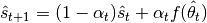M-Step: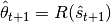Where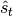is the expected sufficient statistics of the distribution at step,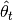is the estimated mixture parameters, function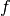maps model parameters and observations to sufficient statistics and function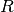maps sufficient statistics to model parameters.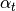is a hyperparemeter called step size. Step size is valued between 0 and 1 and it controls convergence and stability.

At each time step t, sufficient statistics can be generated from a single measurement or multiple measurements in a mini-batch style. Mini-batch processing will improve the stability of the algorithm, and it be set as a hyperparameter.

## Scala¶

Import MultivarateGaussianMixture and functions to generate samples from multivariate gaussian. The mixture weights are sampled using uniform distribution.

import com.github.ozancicek.artan.ml.mixture.MultivariateGaussianMixture
import com.github.ozancicek.artan.ml.SparkFunctions.randMultiGaussian
import org.apache.spark.ml.linalg._
import org.apache.spark.sql.SparkSession
import org.apache.spark.sql.functions._

val spark = SparkSession
.builder
.appName("GMMRateSource")
.getOrCreate
spark.sparkContext.setLogLevel("WARN")
import spark.implicits._

val numStates = 2
val rowsPerSecond = 10
val numMixtures = 3
val minibatchSize = 1

// 3 gaussians for sample generating expression
val dist1 = randMultiGaussian(new DenseVector(Array(1.0, 2.0)), DenseMatrix.eye(2), seed=0)
val dist2 = randMultiGaussian(new DenseVector(Array(10.0, 5.0)), new DenseMatrix(2, 2, Array(4, 2, 2, 4)), seed=1)
val dist3 = randMultiGaussian(new DenseVector(Array(4.0, 4.0)), new DenseMatrix(2, 2, Array(5, 0, 0, 5)), seed=2)

// Mixture weights defined as [0.2, 0,3, 0.5], sample from uniform dist
val weight = rand(seed=0)
val mixture = when(weight < 0.2, dist1).when(weight < 0.5, dist2).otherwise(dist3)


Training multiple models is achieved by mapping samples to models. Each sample can be associated with a different model by creating a ‘key’ column for it and passing it as a parameter with setStateKeyCol. Not specifying any key column will result in training a single model.

Training data is generated using streaming rate source. Streaming rate source generates consecutive numbers with timestamps. These consecutive numbers are binned to simulate sampling for different models.

val inputDf = spark.readStream.format("rate").option("rowsPerSecond", rowsPerSecond).load
.withColumn("mod", $"value" % numStates) .withColumn("stateKey",$"mod".cast("String"))
.withColumn("sample", mixture)

// Set initial values and hyperparams.
val gmm = new MultivariateGaussianMixture()
.setMixtureCount(3)
.setInitialWeights(Array(0.33, 0.33, 0.33))
.setStateKeyCol("stateKey")
.setInitialMeans(Array(Array(3.0, 5.0), Array(6.0, 6.0), Array(7.0, 1.0)))
.setInitialCovariances(Array(Array(1.0, 0.0, 0.0, 1.0), Array(1.0, 0.0, 0.0, 1.0), Array(1.0, 0.0, 0.0, 1.0)))
.setStepSize(0.01)
.setMinibatchSize(minibatchSize)


Run the transformer. The transformer outputs estimates for mixture model parameters for each sample (or minibatch set by setMinibatchSize). Note that due to continuous estimation of the model, inference abstractions compatible with spark ml pipelines are not implemented yet. The output dataframe consists of estimated model.

// Helper udf to pretty print dense vectors & arrays
val floor = (in: Double) => (math floor in * 100)/100
val truncateVector = udf((in: DenseVector) => in.values.map(floor))
val truncateArray= udf((in: Seq[Double]) => in.map(floor))

val query = gmm.transform(inputDf)
.select(
$"stateKey",$"stateIndex", $"mixtureModel.weights",$"mixtureModel.distributions".getItem(0).alias("dist1"),
$"mixtureModel.distributions".getItem(1).alias("dist2"),$"mixtureModel.distributions".getItem(2).alias("dist3"))
.withColumn("weights", truncateArray($"weights")) .withColumn("dist1_mean", truncateVector($"dist1.mean"))
.withColumn("dist2_mean", truncateVector($"dist2.mean")) .withColumn("dist3_mean", truncateVector($"dist3.mean"))
.drop("dist1", "dist2", "dist3")
.writeStream
.queryName("GMMRateSource")
.outputMode("append")
.format("console")
.start()

query.awaitTermination()

/*
-------------------------------------------
Batch: 1
-------------------------------------------
+--------+----------+------------------+------------+------------+------------+
|stateKey|stateIndex|           weights|  dist1_mean|  dist2_mean|  dist3_mean|
+--------+----------+------------------+------------+------------+------------+
|       0|         1|[0.33, 0.33, 0.33]|[2.98, 4.97]|[6.00, 6.00]|[7.02, 1.02]|
|       0|         2|[0.33, 0.33, 0.33]|[2.96, 4.95]|[6.03, 6.00]|[7.03, 1.04]|
|       1|         1|[0.33, 0.33, 0.33]|[2.98, 4.99]|[6.02, 5.99]|[7.00, 1.01]|
|       1|         2|[0.33, 0.33, 0.33]|[2.98, 4.97]|[6.06, 6.00]|[7.03, 1.02]|
+--------+----------+------------------+------------+------------+------------+

-------------------------------------------
Batch: 2
-------------------------------------------
+--------+----------+------------------+------------+------------+------------+
|stateKey|stateIndex|           weights|  dist1_mean|  dist2_mean|  dist3_mean|
+--------+----------+------------------+------------+------------+------------+
|       0|         3|[0.34, 0.33, 0.33]|[2.95, 4.91]|[6.10, 6.04]|[7.03, 1.04]|
|       0|         4|[0.33, 0.34, 0.33]|[2.95, 4.91]|[6.13, 6.03]|[7.04, 1.06]|
|       1|         3|[0.33, 0.33, 0.33]|[2.96, 4.97]|[6.08, 6.00]|[7.02, 1.02]|
|       1|         4|[0.33, 0.33, 0.33]|[2.95, 4.95]|[6.13, 6.01]|[7.06, 1.04]|
+--------+----------+------------------+------------+------------+------------+

-------------------------------------------
Batch: 10
-------------------------------------------
+--------+----------+------------------+------------+------------+------------+
|stateKey|stateIndex|           weights|  dist1_mean|  dist2_mean|  dist3_mean|
+--------+----------+------------------+------------+------------+------------+
|       0|        16|[0.42, 0.45, 0.13]|[2.17, 3.59]|[9.05, 5.64]|[7.57, 1.49]|
|       1|        16|[0.41, 0.30, 0.29]|[2.13, 3.35]|[7.79, 5.61]|[7.71, 1.96]|
+--------+----------+------------------+------------+------------+------------+*/


See examples for the full code

## Python¶

Import MultivarateGaussianMixture and functions to generate samples from multivariate gaussian. The mixture weights are sampled using uniform distribution.

from artan.mixture import MultivariateGaussianMixture
from artan.spark_functions import randnMultiGaussian

from pyspark.sql.types import StringType
from pyspark.sql import SparkSession
import pyspark.sql.functions as F
import numpy as np

spark = SparkSession.builder.appName("GMMRateSource").getOrCreate()
num_states = 2
mps = 10
minibatch_size = 1
num_mixtures = 3

# Define sample generating expression, 3 gaussians and a uniform random for mixture weights

dist1 = randnMultiGaussian(np.array([1.0, 2.0]), np.eye(2), seed=0)
dist2 = randnMultiGaussian(np.array([10.0, 5.0]), np.eye(2)*2 + 2, seed=1)
dist3 = randnMultiGaussian(np.array([4.0, 4.0]), np.eye(2)*5, seed=2)

weight = F.rand(seed=0)
mixture = F\
.when(weight < 0.2, dist1)\
.when(weight < 0.5, dist2)\
.otherwise(dist3)


Training multiple models is achieved by mapping samples to models. Each sample can be associated with a different model by creating a ‘key’ column for it and passing it as a parameter with setStateKeyCol. Not specifying any key column will result in training a single model.

Training data is generated using streaming rate source. Streaming rate source generates consecutive numbers with timestamps. These consecutive numbers are binned to simulate sampling for different models.

input_df = spark.readStream.format("rate").option("rowsPerSecond", mps).load()\
.withColumn("mod", F.col("value") % num_states)\
.withColumn("stateKey", F.col("mod").cast("String"))\
.withColumn("sample", mixture)

eye = [1.0, 0.0, 0.0, 1.0]
gmm = MultivariateGaussianMixture()\
.setMixtureCount(3)\
.setInitialWeights([0.33, 0.33, 0.33])\
.setStateKeyCol("stateKey")\
.setInitialMeans([[3.0, 5.0], [6.0, 6.0], [7.0, 1.0]])\
.setInitialCovariances([eye, eye, eye])\
.setStepSize(0.01)\
.setMinibatchSize(minibatch_size)


Run the transformer. The transformer outputs estimates for mixture model parameters for each sample (or minibatch set by setMinibatchSize). Note that due to continuous estimation of the model, inference abstractions compatible with spark ml pipelines are not implemented yet. The output dataframe consists of estimated model.

truncate_weights = F.udf(lambda x: "[%.2f, %.2f, %.2f]" % (x, x, x), StringType())

truncate_mean = F.udf(lambda x: "[%.2f, %.2f]" % (x, x), StringType())

query = gmm.transform(input_df)\
.select(
"stateKey", "stateIndex", "mixtureModel.weights",
F.col("mixtureModel.distributions").getItem(0).alias("dist1"),
F.col("mixtureModel.distributions").getItem(1).alias("dist2"),
F.col("mixtureModel.distributions").getItem(2).alias("dist3"))\
.withColumn("weights", truncate_weights("weights"))\
.withColumn("dist1_mean", truncate_mean("dist1.mean"))\
.withColumn("dist2_mean", truncate_mean("dist2.mean"))\
.withColumn("dist3_mean", truncate_mean("dist3.mean"))\
.drop("dist1", "dist2", "dist3")\
.writeStream\
.queryName("GMMRateSource")\
.outputMode("append")\
.format("console")\
.start()

"""
-------------------------------------------
Batch: 1
-------------------------------------------
+--------+----------+------------------+------------+------------+------------+
|stateKey|stateIndex|           weights|  dist1_mean|  dist2_mean|  dist3_mean|
+--------+----------+------------------+------------+------------+------------+
|       0|         1|[0.33, 0.33, 0.33]|[2.98, 4.97]|[6.00, 6.00]|[7.02, 1.02]|
|       0|         2|[0.33, 0.33, 0.33]|[2.96, 4.95]|[6.03, 6.00]|[7.03, 1.04]|
|       1|         1|[0.33, 0.33, 0.33]|[2.98, 4.99]|[6.02, 5.99]|[7.00, 1.01]|
|       1|         2|[0.33, 0.33, 0.33]|[2.98, 4.97]|[6.06, 6.00]|[7.03, 1.02]|
+--------+----------+------------------+------------+------------+------------+

-------------------------------------------
Batch: 2
-------------------------------------------
+--------+----------+------------------+------------+------------+------------+
|stateKey|stateIndex|           weights|  dist1_mean|  dist2_mean|  dist3_mean|
+--------+----------+------------------+------------+------------+------------+
|       0|         3|[0.34, 0.33, 0.33]|[2.95, 4.91]|[6.10, 6.04]|[7.03, 1.04]|
|       0|         4|[0.33, 0.34, 0.33]|[2.95, 4.91]|[6.13, 6.03]|[7.04, 1.06]|
|       1|         3|[0.33, 0.33, 0.33]|[2.96, 4.97]|[6.08, 6.00]|[7.02, 1.02]|
|       1|         4|[0.33, 0.33, 0.33]|[2.95, 4.95]|[6.13, 6.01]|[7.06, 1.04]|
+--------+----------+------------------+------------+------------+------------+

-------------------------------------------
Batch: 10
-------------------------------------------
+--------+----------+------------------+------------+------------+------------+
|stateKey|stateIndex|           weights|  dist1_mean|  dist2_mean|  dist3_mean|
+--------+----------+------------------+------------+------------+------------+
|       0|        16|[0.42, 0.45, 0.13]|[2.17, 3.59]|[9.05, 5.64]|[7.57, 1.49]|
|       1|        16|[0.41, 0.30, 0.29]|[2.13, 3.35]|[7.79, 5.61]|[7.71, 1.96]|
+--------+----------+------------------+------------+------------+------------+

"""
query.awaitTermination()


See examples for the full code

 [sEM] Olivier Cappé. Online Expectation-Maximisation. K. Mengersen and M. Titterington and C. P. Robert. Mixtures: Estimation and Applications, Wiley, pp.1-53, 2011. ffhal-00532968f